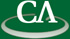Probabilistic Algorithms for the Stable Interpolation of Sparse Approximate Polynomials

Mark Giesbrecht, Symbolic Computation Group, University of Waterloo

Wednesday August 9th, 2006, at 1:30pm in the IRMACS theatre.

```Abstract:

Interpolation of polynomials is a fundamentally important problem in
both numerical and algebraic computing, and has been very well studied
for its mathematical properties.  The usual (dense) representation of
multivariate polynomials can get very large, even at relatively low
degrees, when the number of variables is modest.  Exploiting
sparsity is a key to computing efficiently with them.  Hence, we
consider the problem of sparse interpolation of approximate
multivariate polynomials.   We work in the "black box" model, where
we can evaluate the polynomial at any point, but have no information
on how the values are computed or obtained.  Both the inputs and
outputs of the black-box polynomial will be assumed to have some
error, and all numbers are represented in standard, fixed-precision,
floating point arithmetic.

We present a "probabilistically stable" algorithm which gives a
numerically reliable solution with high probability.  We also note (and
use) the strong similarity between the exact Ben-Or & Tiwari (1988) sparse
interpolation algorithm and the classical Prony's (1795) method for
interpolating a sum of exponential functions, and exploit the
generalized eigenvalue reformulation of Prony's method.  We look at
the numerical stability of our algorithms and the sensitivity of the
solutions, as well as the expected numerical conditioning achieved
through randomization.

This is joint work with Wen-shin Lee and George Labahn.
```